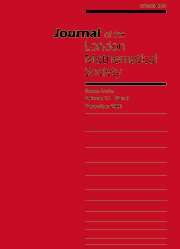HomeJournal of the London Mathematical Society

# sub-laplacians of holomorphic $l^{\lowercase{p}}$-type on exponential solvable groups

Published online by Cambridge University Press:  04 October 2005

## Abstract

let $l$ denote a right-invariant sub-laplacian on an exponential, hence solvable lie group $g$, endowed with a left-invariant haar measure. depending on the structure of $g$, and possibly also that of $l, l$ may admit differentiable $l^p$-functional calculi, or may be of holomorphic $l^p$-type for a given $p{\ne} 2$. ‘holomorphic $l^p$-type’ means that every $l^p$-spectral multiplier for $l$ is necessarily holomorphic in a complex neighbourhood of some non-isolated point of the $l^2$-spectrum of $l$. this can in fact only arise if the group algebra $l^1(g)$ is non-symmetric.

assume that $p{\ne} 2$. for a point $\ell$ in the dual $\frak{g}^*$ of the lie algebra $\frak{g}$ of $g$, denote by $\omega(\ell){=}\ad^*(g)\ell$ the corresponding coadjoint orbit. it is proved that every sub-laplacian on $g$ is of holomorphic $l^p$-type, provided that there exists a point $\ell{\in} \frak{g}^*$ satisfying boidol's condition (which is equivalent to the non-symmetry of $l^1(g)$), such that the restriction of $\omega(\ell)$ to the nilradical of $\frak{g}$ is closed. this work improves on results in previous work by christ and müller and ludwig and müller in twofold ways: on the one hand, no restriction is imposed on the structure of the exponential group $g$, and on the other hand, for the case $p{>}1$, the conditions need to hold for a single coadjoint orbit only, and not for an open set of orbits.

it seems likely that the condition that the restriction of $\omega(\ell)$ to the nilradical of $\frak{g}$ is closed could be replaced by the weaker condition that the orbit $\omega(\ell)$ itself is closed. this would then prove one implication of a conjecture by ludwig and müller, according to which there exists a sub-laplacian of holomorphic $l^1$ (or, more generally, $l^p$) type on $g$ if and only if there exists a point $\ell{\in} \frak{g}^*$ whose orbit is closed and which satisfies boidol's condition.

## Keywords

Type
notes and papers
Information
Journal of the London Mathematical Society , October 2005 , pp. 364 - 390
the london mathematical society 2005

## Access options

Get access to the full version of this content by using one of the access options below.

## Footnotes

this work was supported by the tmr network harmonic analysis and the ihp network harmonic analysis and related problems of the eu.

### Full text views

Full text views reflects PDF downloads, PDFs sent to Google Drive, Dropbox and Kindle and HTML full text views.

Total number of HTML views: 0
Total number of PDF views: 3 *
View data table for this chart

* Views captured on Cambridge Core between September 2016 - 24th January 2021. This data will be updated every 24 hours.

Hostname: page-component-76cb886bbf-wsww6 Total loading time: 0.213 Render date: 2021-01-24T12:25:20.486Z Query parameters: { "hasAccess": "0", "openAccess": "0", "isLogged": "0", "lang": "en" } Feature Flags: { "shouldUseShareProductTool": true, "shouldUseHypothesis": true, "isUnsiloEnabled": true, "metricsAbstractViews": false, "figures": false, "newCiteModal": false }

# Send article to Kindle

Note you can select to send to either the @free.kindle.com or @kindle.com variations. ‘@free.kindle.com’ emails are free but can only be sent to your device when it is connected to wi-fi. ‘@kindle.com’ emails can be delivered even when you are not connected to wi-fi, but note that service fees apply.

Find out more about the Kindle Personal Document Service.

sub-laplacians of holomorphic $l^{\lowercase{p}}$-type on exponential solvable groups
Available formats
×

# Send article to Dropbox

To send this article to your Dropbox account, please select one or more formats and confirm that you agree to abide by our usage policies. If this is the first time you use this feature, you will be asked to authorise Cambridge Core to connect with your <service> account. Find out more about sending content to Dropbox.

sub-laplacians of holomorphic $l^{\lowercase{p}}$-type on exponential solvable groups
Available formats
×

# Send article to Google Drive

To send this article to your Google Drive account, please select one or more formats and confirm that you agree to abide by our usage policies. If this is the first time you use this feature, you will be asked to authorise Cambridge Core to connect with your <service> account. Find out more about sending content to Google Drive.

sub-laplacians of holomorphic $l^{\lowercase{p}}$-type on exponential solvable groups
Available formats
×
×